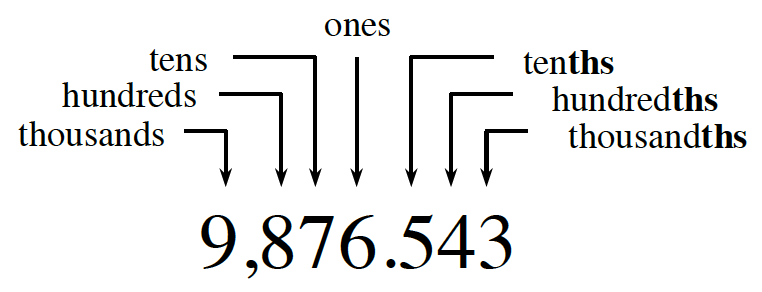### Home > CC1 > Chapter 1 > Lesson 1.1.5 > Problem1-40

1-40.

Round each number to the specified place.

1. $33.54296$ (ten thousandth)

If you need help with place values, refer to this graphic from the Math Notes box in Lesson 1.1.3.To start, find the digit in the place specified by the problem. The ten thousandth place is not shown on the graphic, so you have to find where it should be.

Now, find the digit one place to the right. If it is greater than or equal to $5$, increase the specified digit by $1$.

$33.5430$

2. $307,407$ (thousand)

See part (a).

3. $285.39154$ (hundredth)

$285.39$

4. $6811.09$ (ten)

• See part (a).